##Quadratic Polynomials: The Quadratic Formula.The quadratic formula is nothing else but completing the square once and for all.

#### The derivation of the quadratic formula.

Let us find the roots of the polynomial

ax2+bx+c

by completing the square. First we write the polynomial equation down, and divide both sides by the leading coefficient a: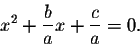Then we transfer the constant term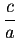to the right side: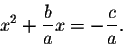We will be using the binomial formula: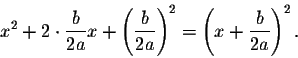Remember: always take half of the term in front of the x. Now we complete the square by adding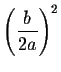on both sides of our equation: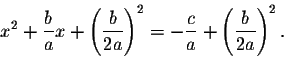We have completed the square! Next we use the binomial formula, and simplify the right side: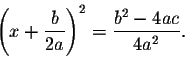Check the simplification on the right side! Taking square roots on both sides, we obtain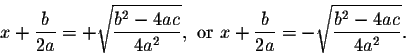Solving for x, we find our two roots: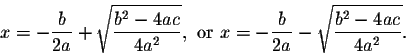We obtain the classical quadratic formula by simplifying and adopting the nasty habit of using the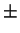notation to save us time:

 The quadratic polynomial ax2+bx+c has the two roots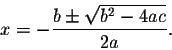This formula is as important and widely used as the Pythagorean Theorem. Teachers do not have mercy on students who do not remember the quadratic formula, unless they can help themselves by completing the square instead!

#### The examples revisited.

Let us check the answers to our three examples in the "completing the square" section.

Find the roots of the polynomial x2+2x-7. In this example a=1, b=2, and c=-7. Using the quadratic formula we see that the roots are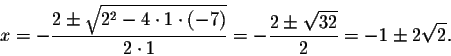Here is our second example again:

2x2+6x+3.

This time a=2, b=6 and c=3, so using the quadratic formula we obtain the roots: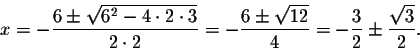Last not least, for the example x2-6x+3, the roots are given by the quadratic formula as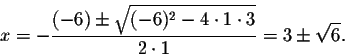#### Exercise 1.

Find the roots of the following polynomials by using both the method of "completing the square", and by the quadratic formula. No answers are provided. If you get the same answers by both methods, I bet you got it right. If the two methods yield different results, you got something wrong, and you have to check them both, or even better: do them again carefully on a new sheet of scratch paper.
• x2+7x-5
• 2x2-4x+1
• 3x2-5x-10
• -2x2+4x+1
•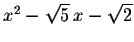[Back] [Next]
[Algebra] [Trigonometry] [Complex Variables]
[Calculus] [Differential Equations] [Matrix Algebra]S.O.S MATHematics home page

Do you need more help? Please post your question on our S.O.S. Mathematics CyberBoard.Helmut Knaust